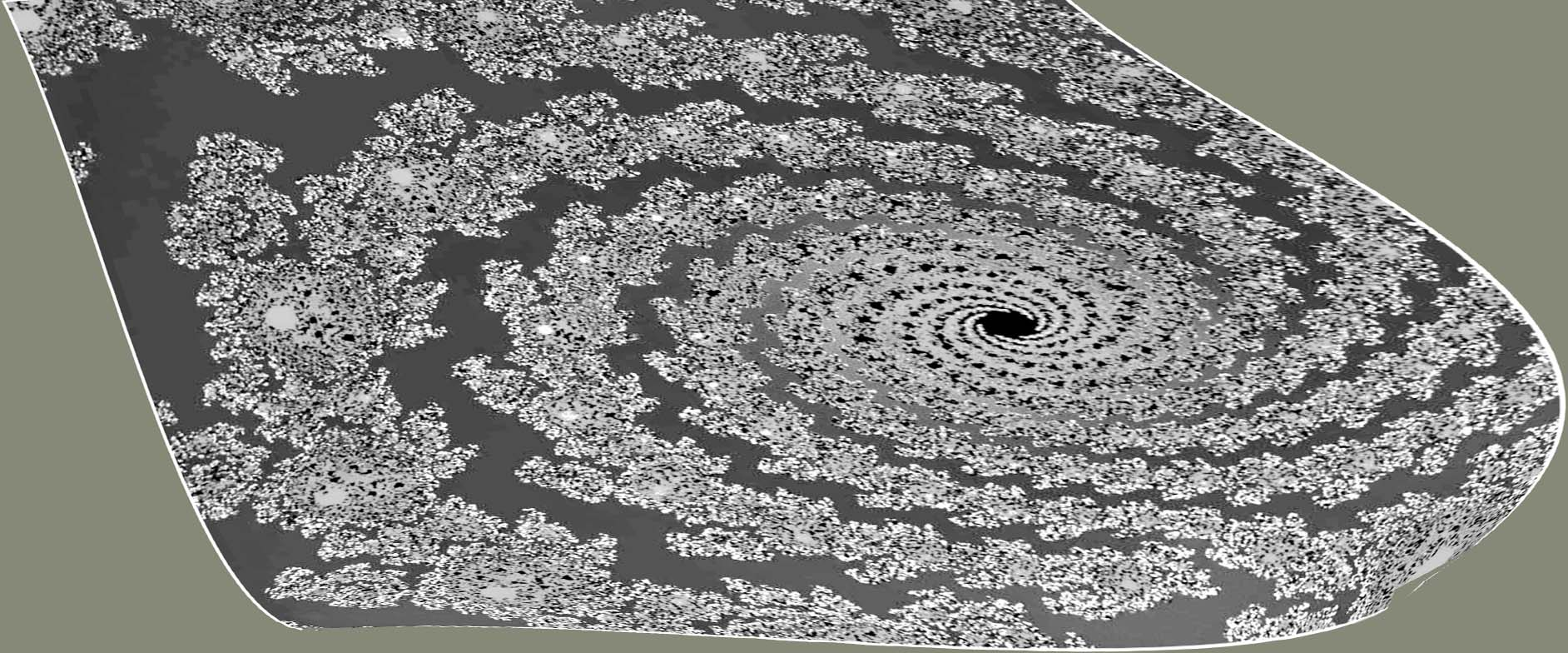# How to plot this fractal

I'm a graphic designer and my client has asked me to use this fractal in a design that I'm working on. As you can see, it's not a very good copy, so I'm trying to see if I can generate a high-resolution version for the project. I've found all sorts of fractal generators that work great and many of them will allow me to enter a specific equation. The trouble is that I'm a designer and not a mathematician! So the question is this: is it plausible to think that this could be reverse engineered, or is that an insane idea? If it's plausible, how would I achieve it?EDIT:

I found the original from the book (below). The values @Carlo Beenakker posted got me very close to the right area. I was able to render and warp the output and achieve what we were looking for! It's not exact, but very close.• At first I was doubting this question was on-topic, but I think there's a very interesting problem here, and it seems like an applied maths kind of thing: how to model observed behaviour, especially the kind of multi-scale self-similarity we have here? Sep 7, 2020 at 0:09
• Are we sure this is not some high-order point in the Mandelbrot set? Sep 7, 2020 at 11:07
• So, I've found out a little more info on the fractal, my client found it in a book titled War in the Age of Intelligent Machines... It seems like a fellow named Robert Devaney is who generated it. Sep 7, 2020 at 15:37

The source info (War in the Age of Intelligent Machines) identifies the fractal as a Julia set, iterates of $$z\mapsto z^2+z_0$$. It has evidently been distorted (warped) to give it a 3D appearance. I played around with a spiral-shaped Julia set I found at pixels.com and could create an image such as this.The Mathematica command
Manipulate[JuliaSetPlot[a- b*I,MaxIterations->n], {a,0,1},{b,0,1},{n,100,1000}] provides a way to find suitable values for $$z_0=a-bi$$ (and the iteration count $$n$$). I find a similar spiral for $$z_0=0.202-0.55\,i$$, $$n=400$$.

If the requirements are:

(a) fractal (image is repeated at small scales); and

(b) clockwise spiral

then the following may be of use:This image is generated by the complex exponential function $$\exp(z)+1+2.9i$$. The resolution can be improved to whatever level you desire (given enough computing power).

Alternatively, if you had a high resolution image of one of the "islands" in your image, then you could make a lattice out of many copies of that, and then apply some sort of a spiral filter which I have seen in some graphics programs like GIMP and maybe Photoshop.

• I think the TS asking about the exact reconstruction of the given fractal. It is obviously, machine-generated, so there should be a formula... But finding it may be enormous mathematical challenge. Sep 7, 2020 at 10:34

It looks like a tiling of some type of julia fractals, then post-distorted with a 'spiral' map. Hence, it seems to fall in the "flame fractal" category. The downside is that there are a lot of parameters to play around with. Also, a reverse image search gave me nothing, so this is not a picture grabbed from the internet.

There is a huge fractal art community at DeviantArt.com - if your client really want something like this, perhaps one can ask one of the artists for a commission?

Side note: I have developed some software to make this type of fractals, so I am rather familiar with the process. Also, in all fractals I generate, I hide the underlying equations as metadata in the image file, so that I can always recreate the fractal (this can be of interest for other mathematicians out there).